# How Many Watts in Kilowatts & Energy Bills Explained…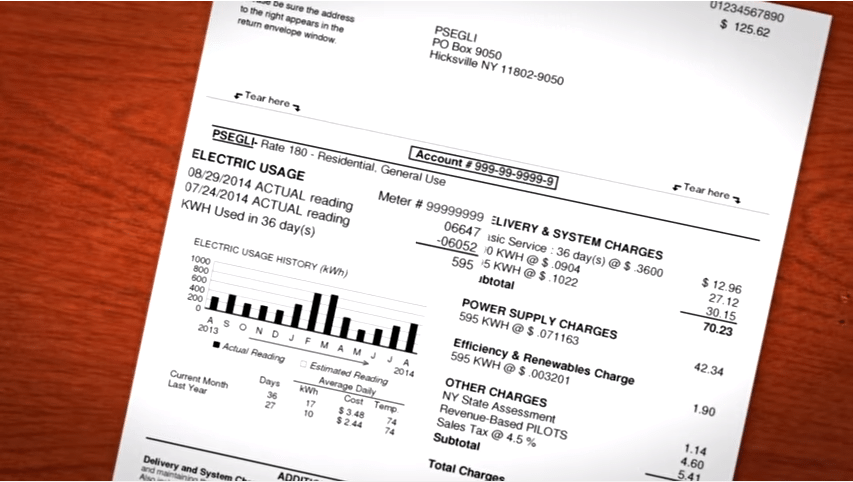When a typical person receives their electricity bill they simply give it a brief glance and then proceed to pay it. While this is all good and fine, most people don’t actually understand what their electric bill means. Specifically, how much electricity they are using. This is an important thing to understand because if you wanted to go about reducing your electricity bill, you need to know where you’re starting from and how much electricity you are consuming in the first place.

understanding-electricity-bill
Since electricity and its related terminology are not things that are very well known to an average person, this can seem a bit intimidating at first. But with a few basic terms known and understood, it is actually quite easy to make sense of your electric bill.

The first and most important thing for you to understand is the term “kilowatt hour.”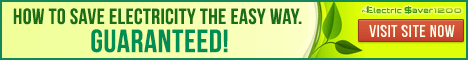## What & How much is a Kilowatt Hour?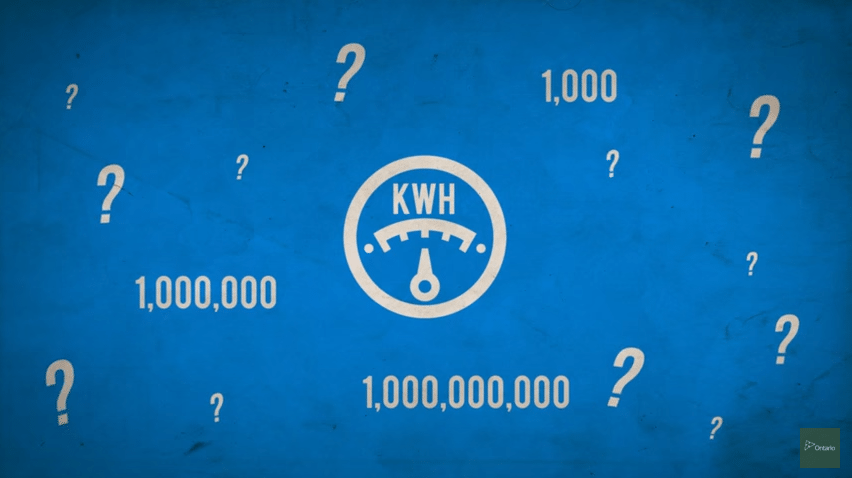A kilowatt hour, abbreviated kWh, is a measurement of energy used. Let’s break this term down. Electrical energy is measured in watts. A good way to get an idea of how much energy a watt is, is to look at a traditional light bulb. A 60 watt light bulb consumes 60 watts of electricity in an hour. The average kilowatt hour cost varies depending on where you live. But, the US National Average price per kilowatt is shown further below.

## How Many Watts In a Kilowatt?

This system of measurement uses the metric system. So a kilowatt is equal to 1000 watts. Now watts and kilowatts are measured with watt hours and kilowatt hours. This is how much electricity is consumed over the given course of an hour.

Let’s go back to the light bulb example to illustrate this. Let’s assume you have a 100 watt light bulb that runs for 10 hours a day. At the end of each day this light bulb would have consumed one kilowatt hour of energy. 100 watts times 10 equals 1 kilowatt. The math is actually pretty simple.

what-is-kilowatt-hour
This is how your power company measures how much electricity you are consuming. If you look at your bill, you will see the total number of kilowatt-hours you consumed.

Now that you know what a kilowatt is you can begin to figure out how much electricity each of your appliances is using.

## How Do Electric Companies Calculate Electric Energy Used?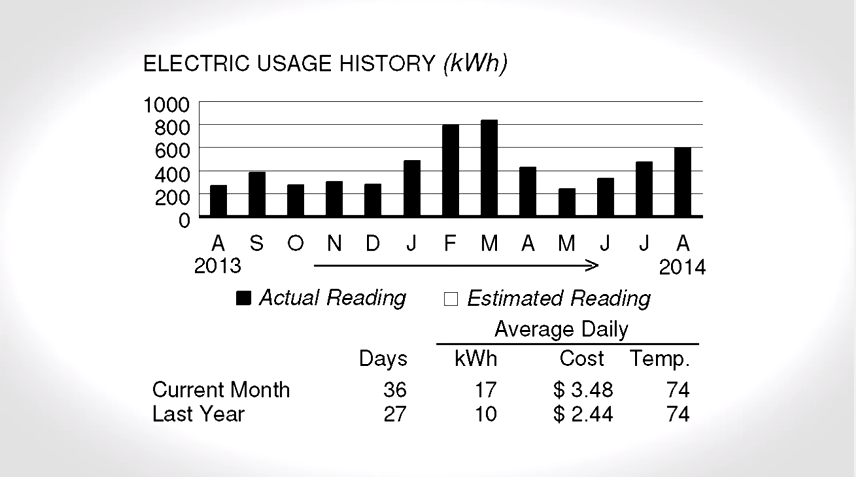By installing a smart electric meter on your property, energy companies can measures all the electricity your property uses. Smart Meters monitor your energy usage easily and remotely. As your power company measures your electrical consumption in terms of kilowatt hours, the next thing to do is to find out how much they charge you for each one. This can vary greatly depending on where you live.

## How Much Does A Kilowatt Hour Cost? Answer: Average in the USA= 12 cents

Depending on where you live in the USA depends on your kilowatt hour cost rate. Hawaii residents have to pay about 24 cents per kilowatt hour which is higher than anywhere else in the country. Other states and regions pay only eight cents per kwh. Central Florida where I live is 11 cents per kilowatt hour. But in Canada, we have been hearing reports of 26 cents per kilowatt hour. And power rates are climbing at an alarming rate. Just last week a gentleman called me from Delaware and said his rates were doubling in the next few years.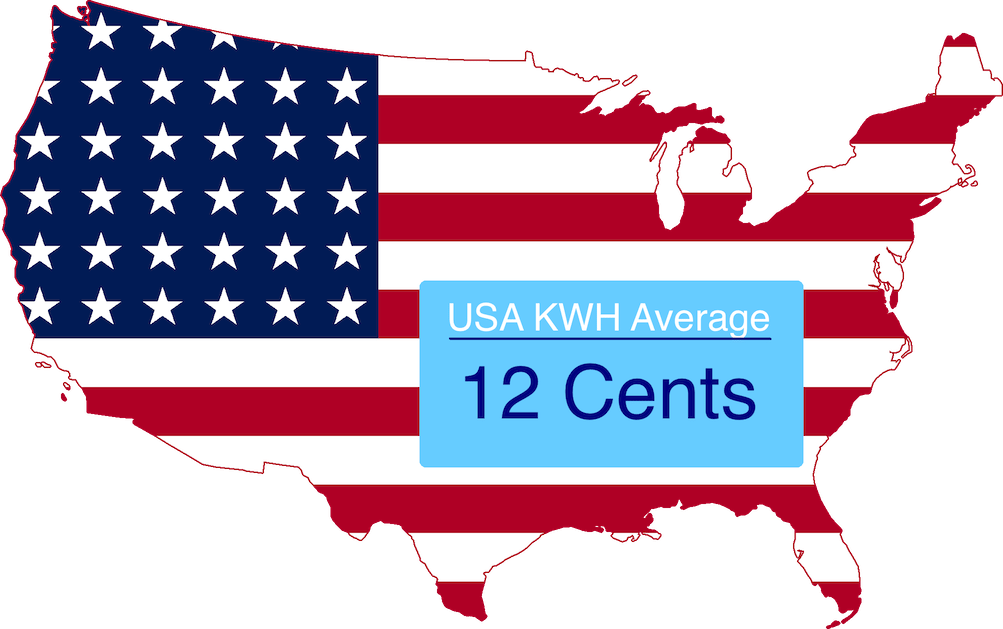The difference can be pretty staggering so you should check your own energy bill to see how much you are being charged.

The average for the nation is 12 cents per kilowatt hour. But again, your rate may vary greatly from this.

Another thing to consider is that your electric company charges you based on how much electricity you use. This means that once you use above a certain amount of electricity, your rate actually goes up.

This information can also be found on your electric bill. If you have any questions about this you can always call your power company and have it explained to you so that you understand how much you are exactly paying for electricity. But don’t expect them to give you tons of great information on using less electricity… that’s like going to a car lot and asking the car salesman, ” Do I need to buy this car?” They are trying to sell cars so of course their answer will always be yes! The power company is there to provide you a commodity, electricity. So asking, ” How can I use less of what you sell? ” is not going to get you many workable answers.

Now that you know what a kilowatt hour is and how the electric company calculates your usage, it’s time to find out exactly WHAT is costing so much in your home or business and HOW to change that immediately so you can stop paying such high energy bills. Let’s start focusing on how you can pay less of your hard earned dollars to your power company. Continue Reading… Why is My Electric Bill So High? Free Tips to Lower Energy Bills Easily. >>